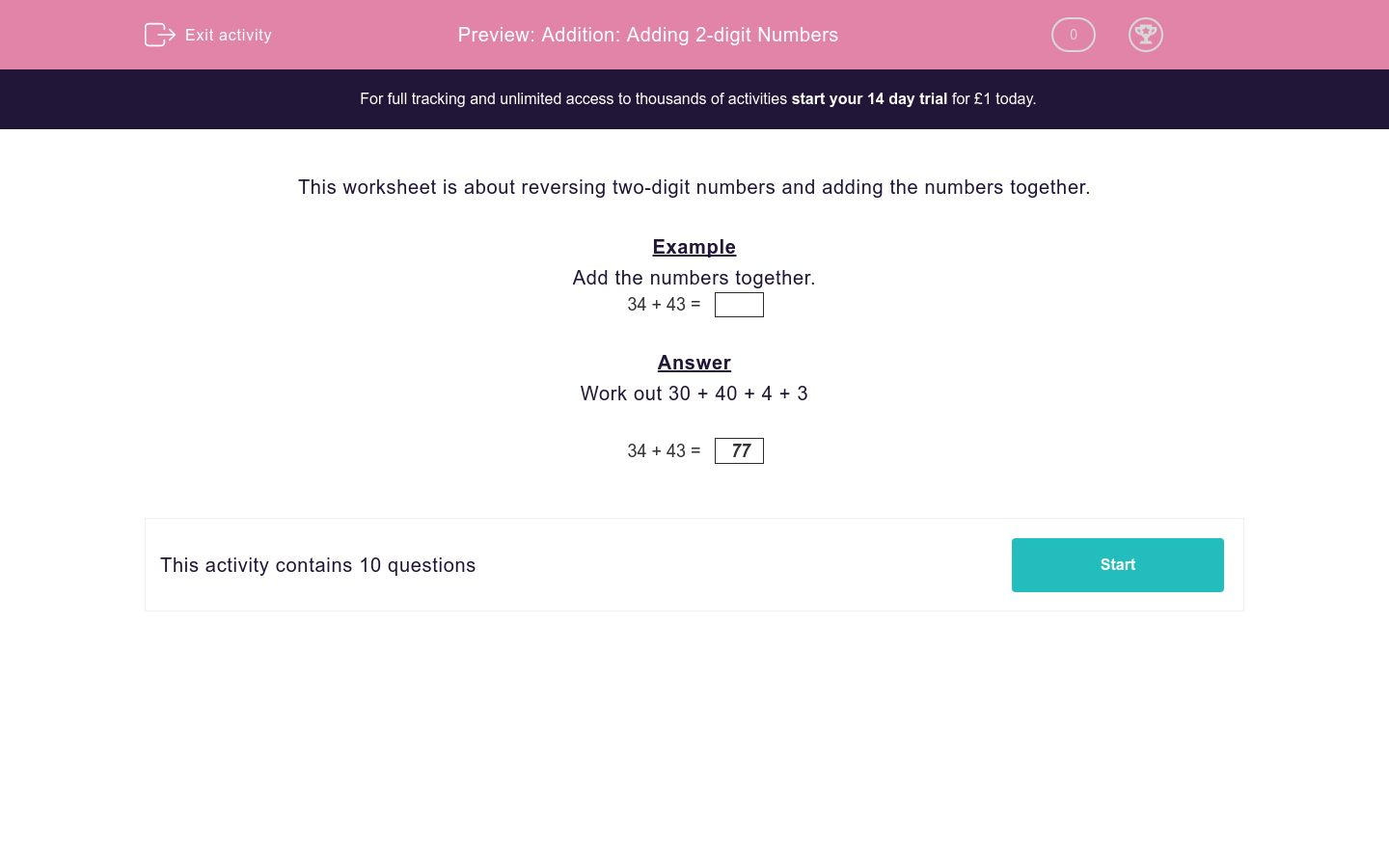### Affordable pricing from £10/month

In this worksheet, students add together two-digit numbers that have been reversed.Key stage:  KS 2

Curriculum topic:   Maths and Numerical Reasoning

Curriculum subtopic:   Mixed Problems

Difficulty level:### QUESTION 1 of 10

This worksheet is about reversing two-digit numbers and adding the numbers together.

Example

34 + 43 =

Work out 30 + 40 + 4 + 3

34 + 43 =
 77

32 + 23 =

35 + 53 =

26 + 62 =

18 + 81 =

25 + 52 =

53 + 35 =

16 + 61 =

15 + 51 =

17 + 71 =

All of your answers have been divisible by 1 and another number.

What is this other number?

• Question 1

32 + 23 =
55
• Question 2

35 + 53 =
88
• Question 3

26 + 62 =
88
• Question 4

18 + 81 =
99
• Question 5

25 + 52 =
77
• Question 6

53 + 35 =
88
• Question 7

16 + 61 =
77
• Question 8

15 + 51 =
66
• Question 9

17 + 71 =
88
• Question 10

All of your answers have been divisible by 1 and another number.

What is this other number?

11
---- OR ----

Sign up for a £1 trial so you can track and measure your child's progress on this activity.

### What is EdPlace?

We're your National Curriculum aligned online education content provider helping each child succeed in English, maths and science from year 1 to GCSE. With an EdPlace account you’ll be able to track and measure progress, helping each child achieve their best. We build confidence and attainment by personalising each child’s learning at a level that suits them.

Get started